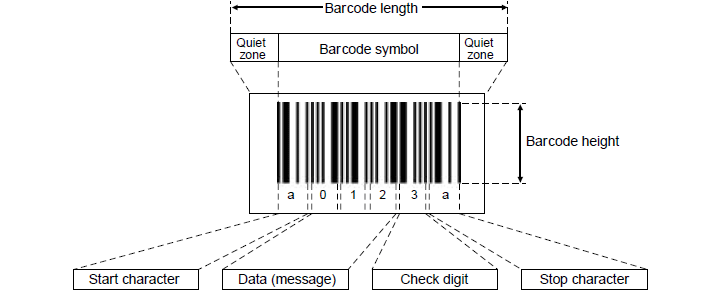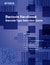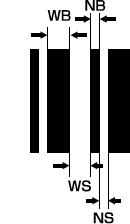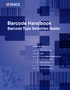1. Home >
2. Basics of Barcodes

# Basics of Barcodes

### Structure of Barcode

The following explains the format of a barcode.#### Quiet zone (margin)

The right and left ends of the barcode symbol.
If the margin is not wide enough, the barcode reader cannot scan the barcode data.
Both the right and left margins should be at least 10 times as wide as the narrow bar width (minimum element width). (See page here for narrow bar width.)

##### One Point

Unless the quiet zones are wide enough, it becomes unstable for the barcode reader to scan the barcode data.

#### Start/stop character

Character to indicate start or end of the data.
Start/stop character varies depending on the type of the barcode. CODE 39 uses “*” and CODABAR uses “a”,“b”,“c” and “d”. (EAN and ITF use, instead of a character, a bar pattern which indicates the start/end of the data.)

#### Data (message)

Bar patterns representing the data (numerical characters, alphabet, etc.) are arranged from left.
The drawing above shows that the data of “012” is demonstrated, with the bar patterns representing 0, 1, and 2 being arranged respectively from the left.

#### Check digit

A numeric value calculated to check for read error. It is appended directly after the barcode data.
(See page here for details)

#### Barcode length

Barcode length defines the total length including the right and left quiet zones.
If the barcode length, includinng quiet zones, does not fit in the scan width, the barcode reader cannot scan the data.

#### Barcode height

It is recommended to make the barcode as high as the printer permits.
If the barcode is not high enough, the laser may deviate from the barcode, causing unstable readings.
It is recommended to keep the height greater than 15 % of the barcode length.

Download### Narrow Bar and Wide Bar

The following explains bars and spaces that are the minimum unit to form a barcode. A barcode is a combination of narrow and wide bars and spaces. Each bar and space are named as listed below:NB Narrow bar Wide bar Narrow space Wide space

Narrow and wide widths are determined at the following ratio:
NB:WB = NS:WS =1:2 to 1:3

##### One Point

If the ratio of narrow and wide is out of the range above, the barcode reader may perform unstable readings.
For preparing a barcode, careful attention shall be made to this ratio. The following ratio is normally recommended for preparing a barcode.
NB:WB = NS:WS =1:2.5 (Recommended ratio)

The width of a narrow bar is a key for selecting a barcode reader.
Narrow bar width is also named "minimum element width".

##### One Point
If the narrow width is small:
The size of a barcode becomes small.
A barcode with many digits can be printed in a given space.
High performance is required for the printer to print a barcode.(laser printer, thermal transfer printer)
If the narrow width is large
The size of a barcode becomes large.
Low performance is allowed for the printer to print a barcode.(dot printer, ink jet printer for FA)

Download### Binary Level and Multiple Level

Barcodes in the categories of CODE 39, CODABAR and ITF consist of bars and spaces that have two steps of narrow and wide widths. It is named the "binary level" barcode.
The ratio between narrow and wide is 1 : 2 to 1 : 3. Some allowance is possible.Barcodes in the categories of EAN and CODE 128 consist of bars and spaces that have four steps of narrow and wide widths. It is named the “multiple level” barcode.
The ratio is 1 : 2 : 3 : 4. Allowance is very limited.##### One Point

"Multiple level" EAN and CODE 128 have four steps of bar width.
If the print quality is not good enough to identify the bar width, reading error tends to occur.
The printers with poor print quality including dot impact printer are not suitable for EAN and CODE 128.

Download### What Is Check Digit?

A check digit is a numeric value calculated to check for read error.
The following explains the check flow and calculation method.Barcode with data “123”and check digit “6”.Check digit is calculated from the data.• The calculated check digit is compared with that appended to the read barcode.
• If they do not match, a read error occurs.
##### One Point
Calculating the check digit
The following explains how to calculate the check digit, taking Modulus 10/3 Weight as an example, which is applied for EAN and ITF.

①Number the code value sequentially starting from the right.
②Multiply each odd numbered value by 3 and each even numbered value by 1.
③Total the multiplied values and then subtract the last digit value of the total value from 10 to get the check digit.Thus, the check digit is calculated to be 7.

Download